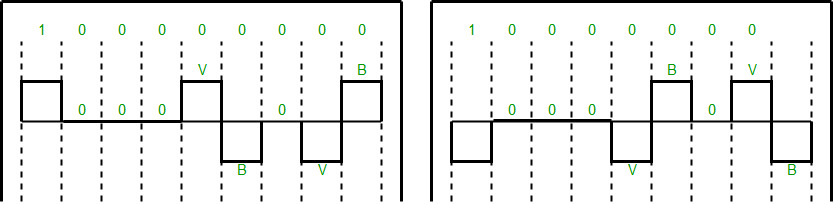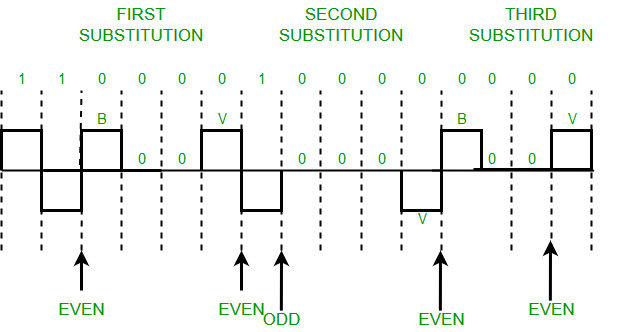# What is Scrambling in Digital Electronics ?

• Difficulty Level : Hard
• Last Updated : 31 May, 2022

A computer network is designed to send information from one point to another. Data that we send can either be digital or analog. Also, signals that represent data can also be digital or analog. Thus to send data by using signals, we must be able to convert the data into signals, this conversion can be Analog to Analog, Analog to Digital, Digital to Analog, or Digital to Digital. Digital to Digital conversion involves three techniques – Line Coding, Block Coding, and Scrambling. Line Coding is always needed, whereas Block Coding and Scrambling may or may not be needed depending upon the need. Scrambling is a technique that does not increase the number of bits and does provide synchronization. The problem with techniques like Bipolar AMI(Alternate Mark Inversion) is that continuous sequence of zero’s create synchronization problems one solution to this is Scrambling.

Prerequisite: Block Coding, Line Coding

There are two common scrambling techniques:

1. B8ZS(Bipolar with 8-zero substitution)
2. HDB3(High-density bipolar3-zero)

B8ZS(Bipolar with 8-zero substitution): This technique is similar to Bipolar AMI except when eight consecutive zero-level voltages are encountered they are replaced by the sequence, “000VB0VB”.

Note:

• V(Violation), is a non-zero voltage which means the signal has the same polarity as the previous non-zero voltage. Thus it is a violation of the general AMI technique.
• B(Bipolar), is also a non-zero voltage level that is in accordance with the AMI rule (i.e., opposite polarity from the previous non-zero voltage).
`Example: Data = 100000000`Note: Both figures (left and right one) are correct, depending upon the last non-zero voltage signal of the previous data sequence (i.e., sequence before current data sequence “100000000”).

HDB3(High-density bipolar3-zero): In this technique, four consecutive zero-level voltages are replaced with a sequence “000V” or “B00V”. Rules for using these sequences:

• If the number of nonzero pulses after the last substitution is odd, the substitution pattern will be “000V”, this helps in maintaining a total number of nonzero pulses even.
• If the number of nonzero pulses after the last substitution is even, the substitution pattern will be “B00V”. Hence even the number of nonzero pulses is maintained again.`Example: Data = 1100001000000000 `

Output explanation: After representing the first two 1’s of data we encounter four consecutive zeros. Since our last substitutions were two 1’s(thus the number of non-zero pulses is even). So, we substitute four zeros with “B00V”.

Note: Zero non-zero pulses are also even.

My Personal Notes arrow_drop_up